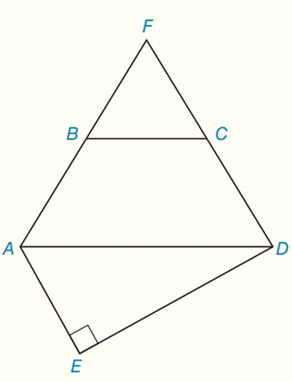Chapter 8.CR, Problem 11CRElementary Geometry For College St...

7th Edition
Alexander + 2 others
ISBN: 9781337614085

Solutions

Chapter
SectionElementary Geometry For College St...

7th Edition
Alexander + 2 others
ISBN: 9781337614085
Textbook Problem

Given: Isosceles trapezoidABCDEquilateral Δ F B C Right Δ A E D BC = 12, AB = 5, and ED = 16 Find: a) A E A F D b) P E A F DTo determine

(a)

To find:

AEAFD

Explanation

Calculation:

Given:

Isosceles trapezoid ABCD Equilateral ΔFBC Right ΔAEDBC = 12, AB = 5, and ED = 16

To find AFBC:

The area of equilateral triangle (all sides congruent) =34s2

Where s is the length of one side of the triangle.

In equilateral ΔFBC, BC (s) = 12 substitute in 34s2

AFBC=34(12)2=34(144)=363

To find AABCD:

AB = 5 and BC = 12

AABCD=12(BC+BC+12(2)AB)(12AB3)=12(12+12+12(2)(5))(12×53)=12(24+12(10))(532)=12(24+5)(532)=14(29×53)=14534

To determine

(b)

To find:

PEAFD

Still sussing out bartleby?

Check out a sample textbook solution.

See a sample solution

The Solution to Your Study Problems

Bartleby provides explanations to thousands of textbook problems written by our experts, many with advanced degrees!

Get Started